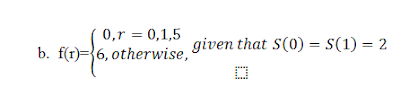## Discrete Structures Question Paper of 3rd Semester CSE74 Download Previous Years Question Paper 5

•Thursday, September 08, 2016
••,
•No comments

December-2003
CS-203/204
DISCRETE STRUCTURES
B.Tech. 3rd Semester-2126
Note: Section –A is compulsory. Attempt any four question from section-B.A attempt any two questions from section –C.
Section –A
1.
a.     What is ternary relation?
b.     Explain the concept of chain.
c.      What are domain, co-domain and image of a function?
d.     Discuss recurrence relations.
e.      What is sub graph?
f.       If. a,b,c are elements of a group G and a*b=c*a, the b=c? explain your answer
g.     What is a ring?
h.     What do you mean by cossets?
i.       How you define degree in a graph?
j.       What is a basic counting principle?

Section-B

2.     Discuss an algorithm of solving order linear homogeneous recurrence relation.

3.     Differentiate between chains and ant chains with the help of suitable example.

4.     State and prove Euler’s formula in connected maps.

5.     How Boolean algebra is applicable in Logic Circuit? Explain with the help of suitable example.

6.     Give an example for simple graph, non-simple, multigraph; directed graphs, weighted graph with diagrams.

Section –C
7.

a.     Solve the recurrence relation S(r)+5(r-1)+6S(r-2)= f(r). where f(r)=b.     What is Quotient ring? Explain with the help of suitable example.

8.     State and prove Lagrange’s theorem on finite groups.

9.     Write short notes on the following;

a.     Eulerian path and circuits.
b.     Linear recurrence relations.

c.      Sum and product rules